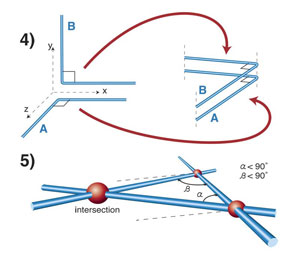# Axioms of GeometryEuclid of Alexandria was a Greek mathematician who lived over 2000 years ago, and is often called the father of geometry. Euclid's book The Elements is the most successful textbook in the history of mathematics, and the earliest known systematic discussion of geometry. In it he laid down the rules of geometry.

He was interested in all the things you could do with a straight edge (a ruler with no marks) and a compass. He came up with his own set of rules that described all the things you could do with these.

1. Given any two points, you can draw a straight line between them (making what’s called a line segment).

2. Any line segment can be made as long as you like (that is, extended indefinitely).

3. Given a point and a line segment starting at the point, you can draw a circle centred on the given point with the given line segment as its radius.##### (Image from Plus)

4. All right angles are equal to each other. (This sounds a bit strange, but was basically Euclid’s rule of how to measure angles and compare them.)

5. The angles of a triangle always add to 180 degrees. (Euclid actually stated it by saying if you draw a line segment across two straight lines and it creates two angles which add to less than two right angles – 180 degrees – then those two straight lines intersect. This rule can be written lots of different ways.)##### (Image from Plus)

These rules describe all the possible things you can draw with a straight edge and compass on a flat piece of paper. By breaking down these straight edge and compass constructions into these fundamental pieces, called axioms, Euclid allowed us to investigate what was possible in this geometry. (You can read about what happens when we break Euclid’s rules here.)

We can do lots of handy things with Euclid’s axioms. Can you figure out how to draw a hexagon using just a straight edge and compass? Can you divide any angle in two? (You can find out more about these constructions here.)

Indeed, these are the tools and methods that stone masons used to create their beautiful decorations and windows in churches and cathedrals. (You can read more here.)